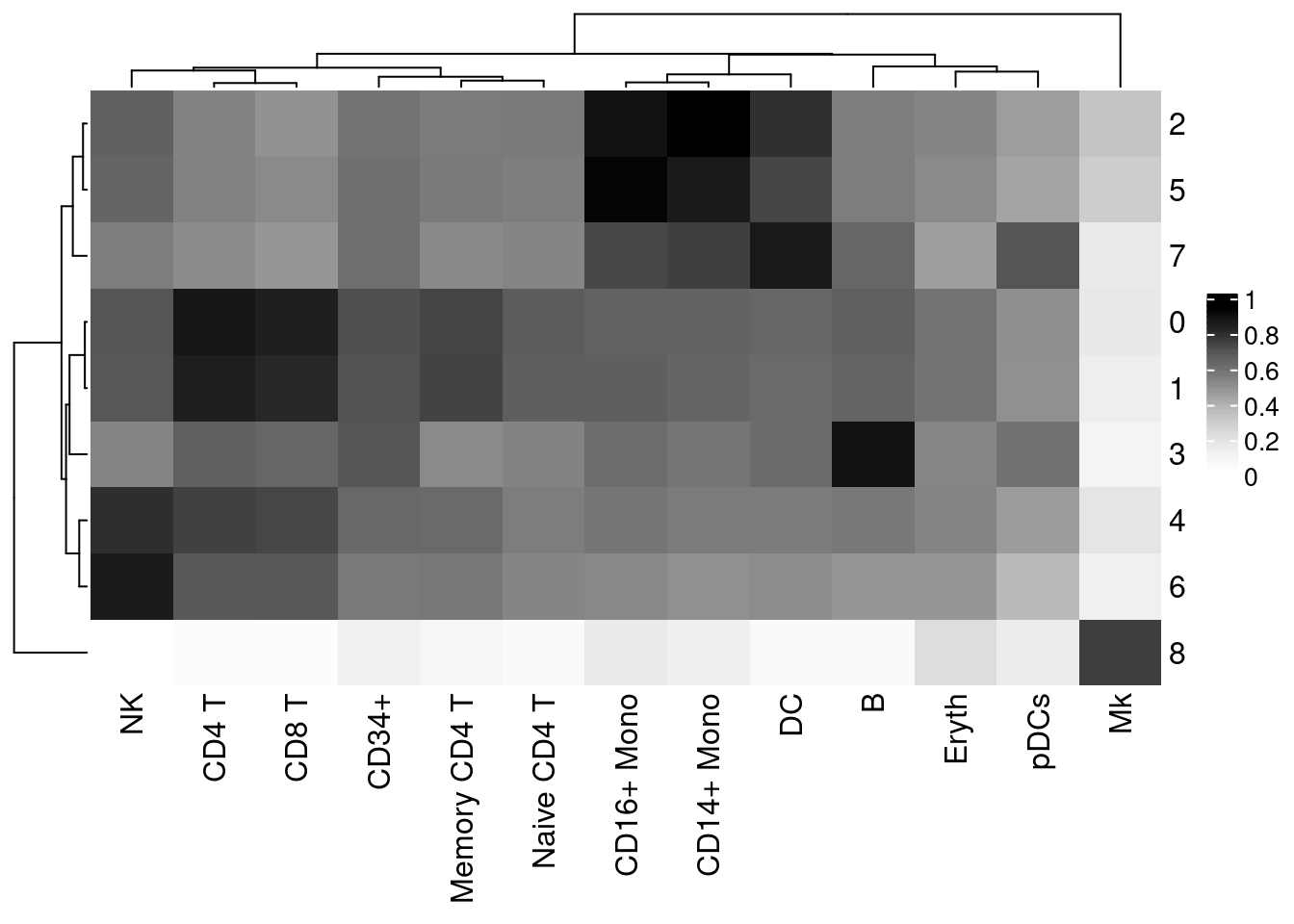# 1 Introduction: Why use `clustifyr`?

Single cell transcriptomes are difficult to annotate without extensive knowledge of the underlying biology of the system in question. Even with this knowledge, accurate identification can be challenging due to the lack of detectable expression of common marker genes defined by bulk RNA-seq, flow cytometry, other single cell RNA-seq platforms, etc.

`clustifyr` solves this problem by providing functions to automatically annotate single cells or clusters using bulk RNA-seq data or marker gene lists (ranked or unranked). Additional functions allow for exploratory analysis of calculated similarities between single cell RNA-seq datasets and reference data.

# 2 Installation

To install `clustifyr` BiocManager must be installed.

``````install.packages("BiocManager")

BiocManager::install("clustifyr")``````

# 3 A simple example: 10x Genomics PBMCs

In this example, we take a 10x Genomics 3’ scRNA-seq dataset from peripheral blood mononuclear cells (PBMCs) and annotate the cell clusters (identified using `Seurat`) using scRNA-seq cell clusters assigned from a CITE-seq experiment.

``````library(clustifyr)
library(ggplot2)
library(cowplot)

# Matrix of normalized single-cell RNA-seq counts
pbmc_matrix <- clustifyr::pbmc_matrix_small

# meta.data table containing cluster assignments for each cell
# The table that we are using also contains the known cell identities in the "classified" column
pbmc_meta <- clustifyr::pbmc_meta``````

# 4 Calculate correlation coefficients

To identify cell types, the `clustifyr()` function requires several inputs:

• `input`: an SingleCellExperiment or Seurat object or a matrix of normalized single-cell RNA-seq counts
• `metadata`: a meta.data table containing the cluster assignments for each cell (not required if a Seurat object is given)
• `ref_mat`: a reference matrix containing RNA-seq expression data for each cell type of interest
• `query_genes`: a list of genes to use for comparison (optional but recommended)

When using a matrix of scRNA-seq counts `clustifyr()` will return a matrix of correlation coefficients for each cell type and cluster, with the rownames corresponding to the cluster number.

``````# Calculate correlation coefficients for each cluster (spearman by default)
vargenes <- pbmc_vargenes[1:500]

res <- clustify(
input = pbmc_matrix, # matrix of normalized scRNA-seq counts (or SCE/Seurat object)
metadata = pbmc_meta, # meta.data table containing cell clusters
cluster_col = "seurat_clusters", # name of column in meta.data containing cell clusters
ref_mat = cbmc_ref, # matrix of RNA-seq expression data for each cell type
query_genes = vargenes # list of highly varible genes identified with Seurat
)

# Peek at correlation matrix
res[1:5, 1:5]
#>           B CD14+ Mono CD16+ Mono     CD34+     CD4 T
#> 0 0.6563466  0.6454029  0.6485863 0.7089861 0.8804508
#> 1 0.6394363  0.6388404  0.6569401 0.7027430 0.8488750
#> 2 0.5524081  0.9372089  0.8930158 0.5879264 0.5347312
#> 3 0.8945380  0.5801453  0.6146857 0.6955897 0.6566739
#> 4 0.5711643  0.5623870  0.5826233 0.6280913 0.7467347

# Call cell types
res2 <- cor_to_call(
cor_mat = res,                  # matrix correlation coefficients
cluster_col = "seurat_clusters" # name of column in meta.data containing cell clusters
)
res2[1:5, ]
#> # A tibble: 5 x 3
#> # Groups:   seurat_clusters 
#>   seurat_clusters type           r
#>   <chr>           <chr>      <dbl>
#> 1 3               B          0.895
#> 2 2               CD14+ Mono 0.937
#> 3 5               CD16+ Mono 0.929
#> 4 0               CD4 T      0.880
#> 5 1               CD4 T      0.849

# Insert into original metadata as "type" column
pbmc_meta2 <- call_to_metadata(
res = res2,                     # data.frame of called cell type for each cluster
metadata = pbmc_meta,           # original meta.data table containing cell clusters
cluster_col = "seurat_clusters" # name of column in meta.data containing cell clusters
)``````

To visualize the `clustifyr()` results we can use the `plot_cor_heatmap()` function to plot the correlation coefficients for each cluster and each cell type.

``````# Create heatmap of correlation coefficients using clustifyr() output
plot_cor_heatmap(cor_mat = res)``````# 5 Plot cluster identities and correlation coefficients

`clustifyr` also provides functions to overlay correlation coefficients on pre-calculated tSNE embeddings (or those from any other dimensionality reduction method).

``````# Overlay correlation coefficients on UMAPs for the first two cell types
corr_umaps <- plot_cor(
cor_mat = res,                     # matrix of correlation coefficients from clustifyr()
metadata = pbmc_meta,              # meta.data table containing UMAP or tSNE data
data_to_plot = colnames(res)[1:2], # name of cell type(s) to plot correlation coefficients
cluster_col = "seurat_clusters"    # name of column in meta.data containing cell clusters
)

plot_grid(
plotlist = corr_umaps,
rel_widths = c(0.47, 0.53)
)``````